Physical Chemistry : Real and Ideal Gases

Example Questions

Example Question #51 : Physical Chemistry

Given the ideal gas law:$\ P = \rho \frac{R}{M}T$

Where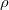is density,is pressure,is the gas constant,is molar mass, and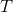is temperature.

Based on the Ideal gas law, which of the following are true?

I. Pressure and volume are inversely proportional

II. Pressure and density are inversely proportional

III. Pressure and temperature are directly proportional

IV. Density and temperature are inversely proportional

V. R and M are inversely proportional

II, III, V

I, III

I, III, IV

III, IV, V

I, II, III, IV, V

I, III, IV

Explanation:

Condition I is true. Pressure and volume are inversely proportional. The ideal gas law with volume can be rederived to show this:

We introduce an expression for moles:$n = {\frac{m}{M}}$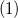Whereis moles,is mass, andis molar mass in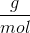Rearranging (1) to solve forand plugging into the following:$\ P = \rho \frac{R}{M}T$

We get: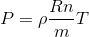(2)

Finally, we use the following for density, whereis volume, and plug into (2)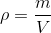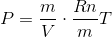The masses cancel out and we have the ideal gas law expressed with volume and moles.

Condition II is false. It is clear from the equation that pressure and density are directly proportional.

Condition III is true because it is clear from the equation that temperature and pressure are directly proportional.

Condition IV is true because density and temperature are on the same side of the equation in the numerator, so the must be inversely proportional.

Condition V is false because the ideal gas constant and molar mass can be rearranged to be on opposite sides of the equation and in the numerator.

Example Question #52 : Physical Chemistry

Under which conditions do gases deviate from ideality?

I. Low temperature

II. High temperature

III. Low pressure

IV. High pressure

V. Gases always behave ideally

I and III

II and III

I and IV

II and IV

V only

I and IV

Explanation:

Gases deviate from ideal conditions at low temperature and high pressure. This because the postulates of the kinetic molecular theory of gasses ignore the volume of the molecules and all interactions between gas molecules. However, neither are true for real gasses. As the temperature increases, the kinetic energy of the particles can overcome the intermolecular forces of attraction or repulsion between the molecules. At high pressures, and subsequently low volume, the distance between molecules becomes shorter, and therefore intermolecular forces become significant. So, ideal conditions are when the distance between molecules is great, and the energy each molecule has is much greater in relative magnitude to the intermolecular forces. This occurs when the pressure is low, and the temperature is high.

Example Question #53 : Physical Chemistry

Given the van der Waals Equation: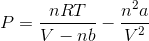Which statement accurately identifies and explains the corrections that van der Waals made to the ideal gas law, where the first correction is the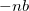term and the second correction is the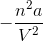term?

The first correction corrects for volume of molecules by increasing volume as a function of the number of molecules, and the second correction corrects for molecular attraction by decreasing pressure as volume increases and number of molecules increases.

The first correction corrects for volume of molecules by decreasing volume as a function of the number of molecules, and the second correction corrects for molecular attraction by decreasing pressure as volume decreases and number of molecules increases.

The first correction corrects for volume of molecules by increasing volume as a function of the number of molecules, and the second correction corrects for molecular attraction by increasing pressure as volume increases and number of molecules increases.

The first correction corrects for volume of molecules by decreasing volume as a function of the number of molecules, and the second correction corrects for molecular attraction by increasing pressure as volume increases and number of molecules increases.

The first correction corrects for volume of molecules by increasing volume as a function of the number of molecules, and the second correction corrects for molecular attraction by increasing pressure as volume decreases and number of molecules increases.

The first correction corrects for volume of molecules by decreasing volume as a function of the number of molecules, and the second correction corrects for molecular attraction by decreasing pressure as volume decreases and number of molecules increases.

Explanation:

All the statements say similar things, but only one correctly identifies the resulting change to volume and pressure. Let's break things down by the correction.

Correction 1:

The correction decreases the volume, since it is subtracting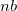from the overall volume. As n (or the number of molecules) goes up, volume will go down.

Correction 2:

Since we are subtracting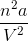from the overall pressure, we are correcting for molecular attraction by decreasing pressure. We are doing this with a termthat gets larger whenincreases and and gets larger asdecreases.

All Physical Chemistry Resources